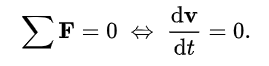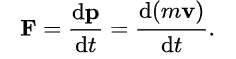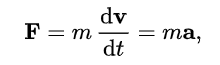# AP Board Class 9 Physical Science Chapter 3 Laws Of Motion

In chapter 2, students are already introduced to the fundamentals of motion, its definition and all its related concepts. However, in chapter 3 of Andhra Pradesh board Class 9 Physical Science textbook, students will expand their knowledge about this concept and learn about the three important laws of motion, which have been formulated by Sir Issac Newton, who is stated to be one of the most influential and widely known scientists in the world.

## Newton’s First Law

As per Newton’s First Law of Motion, a body continues to be in the state of rest or of uniform motion until a net force acts on it. The first law can be represented as,### Newton’s Second Law

Newton’s Second Law of Motion states that the rate of change of momentum of an object is directly proportional to the net force acting on it. The change takes place in the direction of the net force.### Newton’s Third Law

Newton’s Third Law of Motion states if an object exerts some amount of force on another object, the second object also exerts an equal amount of force on the first one but in a completely opposite direction.

Some Related Terms

• The tendency of objects to resist a change in their state of rest or of uniform motion is called inertia. The mass of an object is a measure of inertia. SI unit of mass is Kilogram (kg).
• The linear momentum of a body is the product of its mass and velocity. p = mv

Students can go through some of the chapter questions given below to gain a clearer understanding of the topics.

Question 1: Which one has more inertia object with 5kg or 15kg?

Answer: Object with 15 kg will have more inertia. The reason is that inertia is directly proportional to the mass of an object. Further, we need more force to move the 15 kg object as compared to the 5 kg one.

Question 2: Define Impulsive forces.

Answer: Impulsive forces are forces that are exerted over a limited time. Impulsive forces are mainly of very high magnitude.

Question 3: What is Newton’s First Law of Motion?

Answer: “Every object will remain at rest or in a state of uniform motion unless compelled to change its state by the action of a net force.”

Question 4: Define Inertia of an Object.

Answer: Inertia is a property of matter that resists changes in its state of motion or rest, and it depends on the mass of the object.  The mass of an object is considered as the measure of inertia. We know that the SI unit of mass is ‘kg.’

Keep visiting BYJU’s to access various Andhra board Science study materials, including syllabus, exam timetable and more.